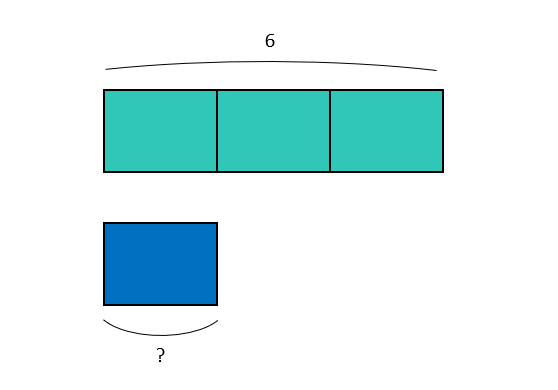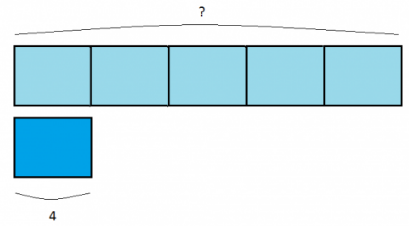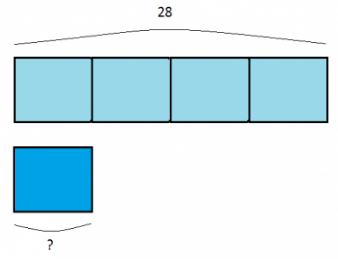Smartick is a fun way to learn math!Nov25

# Singapore Bar Models for Multiplication and Division

Singapore Math Bar Models are a very useful, visual method to teach simple word problems. Some weeks ago we learned how to use the Singapore Bar Models applied to fractions and today we are going to use it for multiplication and division problems.

We have two quantities where one is a multiple of the other. Assuming that we’re not multiplying by 1 (we won’t be talking about this case today), we’ll have one larger quantity and one smaller one. The relationship between these two quantities is quantitative and is called a multiple. How can we find this number? By dividing:

larger quantity / smaller quantity = multiple

A multiple is a number that we refer to when we say “twice as many as”, “three times as many as”…

For example:

Sam and John left on a field trip. Sam took 6 pictures and John took 3. How many more pictures did Sam take than John?

First, we need to determine which is the big quantity and which is the small one:

Larger quantity: 6

Smaller quantity: 3

Then we draw the Singapore bar model to see what quantity fits 3 times into 6:So, the multiple is 6 / 3 = 2.

In other words, Sam took twice as many pictures as John.

Let’s imagine a new problem where we need to calculate the larger quantity having been given the smaller quantity. So, we’ll have to:

Smaller quantity x multiple = Larger quantity

With the Singapore Math Bar Model it’s simple, as we can see in the following example:

Joe and Claudia are rolling dies. Joe won 4 times and Claudia won 5 times more than Joe. How many times did Claudia win?

Smaller quantity: 4

Multiple: 5

The corresponding Singapore Bar Model is:So then, the larger quantity is 4 x 5 = 20. Claudia won 20 times.

In this example, each bar unit represents 4 of Joe’s wins. By repeating that unit 5 times as the problem says, it’s easy to see what we have to multiply.

Lastly, let’s imagine that we have the larger quantity and the multiple and that we want to calculate the smaller quantity. We’ll have to:

Larger quantity / multiple = smaller quantity

Just like before, it’ll be much easier to envision the problem using an example and with the Singapore Bar Model:

Last week, Joe competed in a cooking contest and, although he didn’t win, he earned 28 points, 4 times as many as the participant who came in last place. How many points did that participant earn?

Larger quantity: 28

Multiple: 4

The corresponding Singapore Bar Model is:We can see that the smaller quantity is 28 / 4 = 7.

The participant who came in last place earned 7 points.

In this example, we don’t know how much each unit represents, but we can see that the total quantity is 28 and we want to see how much of the unit remains if we divide it by 4 parts.

Now, you know how to use the Singapore Bar Model in multiplication and division problems!

In those other blog entries, you can practice multiplication and division:

If you want to keep learning lots and lots of math, sign onto Smartick and make an account!

Fun is our brain’s favorite way of learning
Diane Ackerman
Smartick is a fun way to learn math
• 15 fun minutes a day
• Millions of students since 2009• Craig ANDREWSMay 09 2018, 5:10 PM

In your first example, I find the Bar Model diagram confusing. The bar is broken in the middle (in the 4th frame).

Are you trying to demonstrate a roll of film showing the frames/pictures taken ??
In brief, this is not clear to me nor my students. I suggest that Bar model be redone in a clearer way.

OR please explain what is meant in this example.